Home > INT3 > Chapter 7 > Lesson 7.1.4 > Problem7-46

7-46.
1.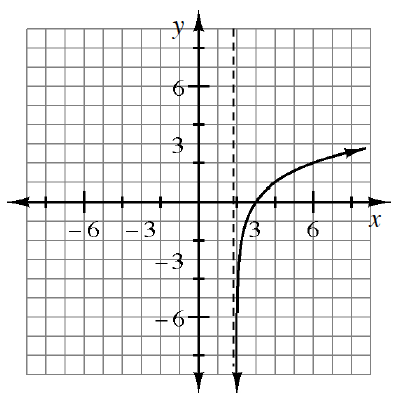Consider the graph at right. Homework Help ✎

1. What is the parent function for this graph?

2. What is the equation of the vertical asymptote?

3. Write a possible equation for this graph.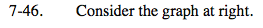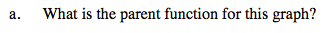y = log(x)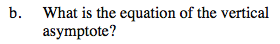Where does the vertical asymptote cross the x-axis?

x = 2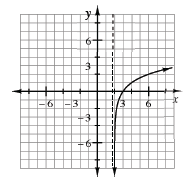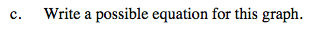How is the graph shifted?

This equation is of the form y = logb(x − 2). Notice that the point (6, 2) is on the graph. Use this point to determine the base.

y = log2(x − 2)